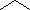×

全部
• 全部
• 黑色
• 脸红
• 奶油
• 白金计划
• 裸体
• 白色

全部
• 全部
• 内衣
• 内裤
• 吊带
• 机构
• 睡衣
• 礼服
• 上衣
• 连衣裙
• 钢圈
• 满满一杯
• 软垫
• 软胸罩
• 高顶点
• 跳水
• Dd +杯
• 真丝文胸
• 纯属
• 完整的内裤
• 丁字裤
• 开放

所有
• 所有
• 32B
• 32C
• 32D
• 32DD
• 32E
• 34B
• 34C
• 34D
• 34DD
• 34E
• 36B
• 36C
• 36D
• 36DD
• 36E
• XS
• S
• M
• L
• XL
• S / M
• M / L

﻿